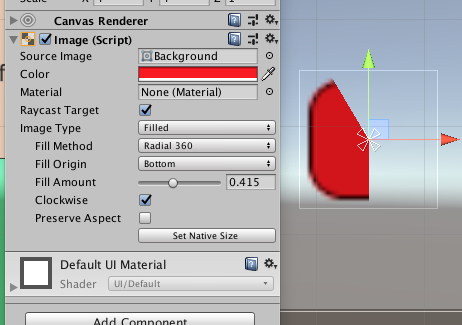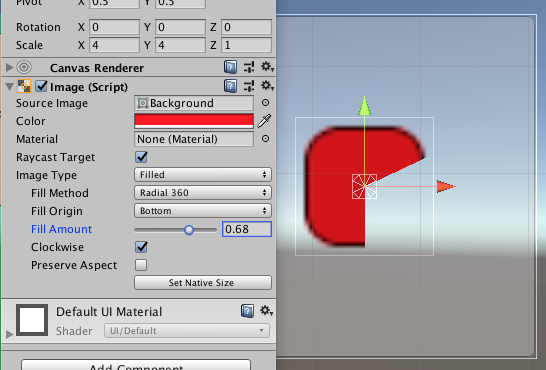# Creating a Circular Progressbar / Timer

I'd like to know how to make a circular progressbar or timer-type effect. Basically, given a value [0..1], it will "fill in" a portion of a circle on the GUI that circularly represents the value. So if I give it 0.33, it should fill in 1/3 of the circle, and so on. These types of GUI elements are useful for timers and that sort of thing.

I really can't think of a way to do this off the top of my head, so if anyone can either post some code or point me in the right direction, that would be awesome. :)

The easiest way is to use an alpha gradient texture:Which is used as a mask in an alpha test (transparent cutout) shader. You change the _Cutoff property to indicate which percentage should be used, ranging from 0..1. This code would make the bar empty when the mouse pointer is on the right side of the screen, going up to full as you move the mouse pointer to the left side (see demo):

``````function Update () {
renderer.material.SetFloat("_Cutoff", Mathf.InverseLerp(0, Screen.width, Input.mousePosition.x));
}

``````

In the demo, there are three textures; one for the regular graphics (using normal alpha for nice anti-aliasing), one for the green health bar (using alpha cutoff), and a solid red behind the first two that shows through where there's no green. The non-alpha part of the health bar texture is just a block of solid green, although it doesn't have to be...you can make it look like whatever you want of course.A unitypackage of this is available here.

FYI this can be done very easily with the new `UI`. All you need is a sprite with a circular texture (I’ve provided one). Add an Image object to your scene, and the provided sprite as the Source Image, and set the Image Type to Filled.

You can access the fill amount via scripting by getting a reference to the Image component (make sure to add import or add a using statement for UnityEngine.UI).

Enjoy

2019 …

This is now built in to Unity. Image → “Filled” → Radial 360.

Just change “Fill Amount” ( `.fillAmount` in script).Use any image you wish.

A more mathematical approach to this problem is:

Render lines that correspond to the part of your circle you want each time.

Here’s what I 've done using the GL class:

``````using UnityEngine;
using System.Collections;

public class timer : MonoBehaviour
{
//assign a material for your lines
public Material lineMat;
public float time;
private float _timer;
private float length=0.5f;
private Vector3 timerpos;
float x,y,delta;

void Start()
{
//Initialize timerPos here
delta = 0.02f;
CreateMat ();
}

void Update()
{
if (time - _timer > 0)
_timer += Time.deltaTime;
}

void OnPostRender()
{
if (time - _timer > 0)
{
for (float angle = 0; angle <(_timer/time) * 2*Mathf.PI; angle+=delta)
{
x = length * Mathf.Cos (-angle + Mathf.PI / 2 );
y = length * Mathf.Sin (-angle + Mathf.PI / 2 );
lineMaterial.SetPass(0);
GL.Begin (1);
GL.Color (Color.white);
GL.Vertex3 (timerpos.x, timerpos.y, 0);
GL.Vertex3 (timerpos.x + x,timerpos.y + y, 0);
GL.End ();
}
}
}
}
// end
``````

For anti-clockwise

``````x = length * Mathf.Cos (angle + Mathf.PI / 2 );
y = length * Mathf.Sin (angle + Mathf.PI / 2 );
``````

The `Mathf.PI / 2` is added so that the timer starts from 12 'o clock

P.S I am not sure if this code is so fast or not but it gets the work done

Unity has since added the Sprite Mask component which works very similarly to the alpha cutout shader @Eric5h5 posted. Unity - Manual: Sprite Masks

This can be done without any need to code at all by combining the image settings described by Noob_Vulcan with a regular Ui Slider.

You can then use the normal slider controls (maxValue, value) and the fill image will automatically fill in a circular shape.